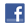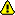New account
4 million accounts created!

• Home
• Report a bug

•

Learn Italian > Italian lessons and exercises > Italian test #21884> Other Italian exercises on the same topic: Numbers [Change theme] > Similar tests: - Numbers - Numers - Numbers - Numbers from 0 to 20 - Numbers from 0 to 20 - Numbers 1-30 - Ordinal numbers - Numbers > Double-click on words you don't understandTwitter Share
Italian exercise "Additions" created by bridg with The test builder. [More lessons & exercises from bridg]Please log in to save your progress.

1.+=

2.=

3.+=

4.++=

5.+=

6.+++7.+=

8.=

9.++=

10.+=

11.++=

12.+=

13.+++=

14.++=

15.++=

16.+++=

17.+++=

18.++=

19.+++=

20.++=

End of the free exercise to learn Italian: Additions
A free Italian exercise to learn Italian.
Other Italian exercises on the same topic : Numbers | All our lessons and exercises